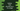# Find all prime numbers in a range in python## Find all prime numbers in a range in python:

In this post, we will learn how to find all prime numbers in a range in Python. The program will take the first and last number of the range and print out all prime numbers in that range.

### What is a prime number:

A positive number, which is larger than 1 and its factors are only 1 and the number itself is called a prime number.

For example, 2, 3, 5, 7, 11 etc. are prime numbers. Because we don’t have only factors only 1 and the number itself. But, 4, 6, 8 etc. are not prime.

### Find prime numbers in a range:

To find if a number is prime or not, we can check if any number from 2 to square root of the number can divide the number or not. We don’t have to check all the numbers from 2 to that number. We can check up to the square root because if a * b = num then we can’t have both a and b greater than square root of num.

Let’s write one program to print all prime numbers from 1 to 100:

``````import math

for num in range(1, 101):
if num > 1:
for i in range(2, int(math.sqrt(num)) + 1):
if(num % i) == 0:
break;
else:
print(num)``````

It will print the prime numbers below 100:

``````2
3
5
7
11
13
17
19
23
29
31
37
41
43
47
53
59
61
67
71
73
79
83
89
97``````

### By taking user input for the range:

``````import math

start = int(input('Enter the start number : '))
end = int(input('Enter the end number : '))

for num in range(start, end+1):
if num > 1:
for i in range(2, int(math.sqrt(num)) + 1):
if(num % i) == 0:
break;
else:
print(num)``````

It will print same output:

``````Enter the start number : 1
Enter the end number : 10
2
3
5
7``````

### Using a different method :

We can also create one different method to find out if a number is prime or not :

``````import math

def isPrime(num):
for i in range(2, int(math.sqrt(num)) + 1):
if(num % i) == 0:
return False;
else:
return True

start = int(input('Enter the start number : '))
end = int(input('Enter the end number : '))

for num in range(start, end+1):
if num > 1:
if isPrime(num) == True:
print(num)``````

It gives similar output:

``````Enter the start number : 1
Enter the end number : 10
5
7
9``````